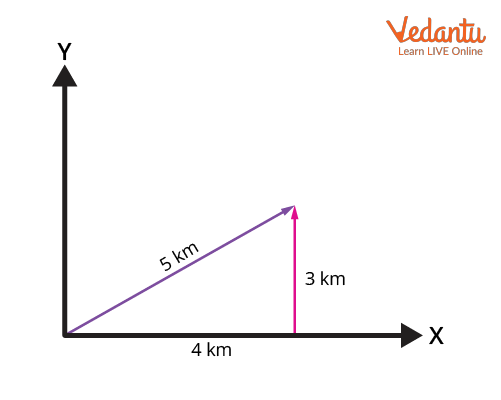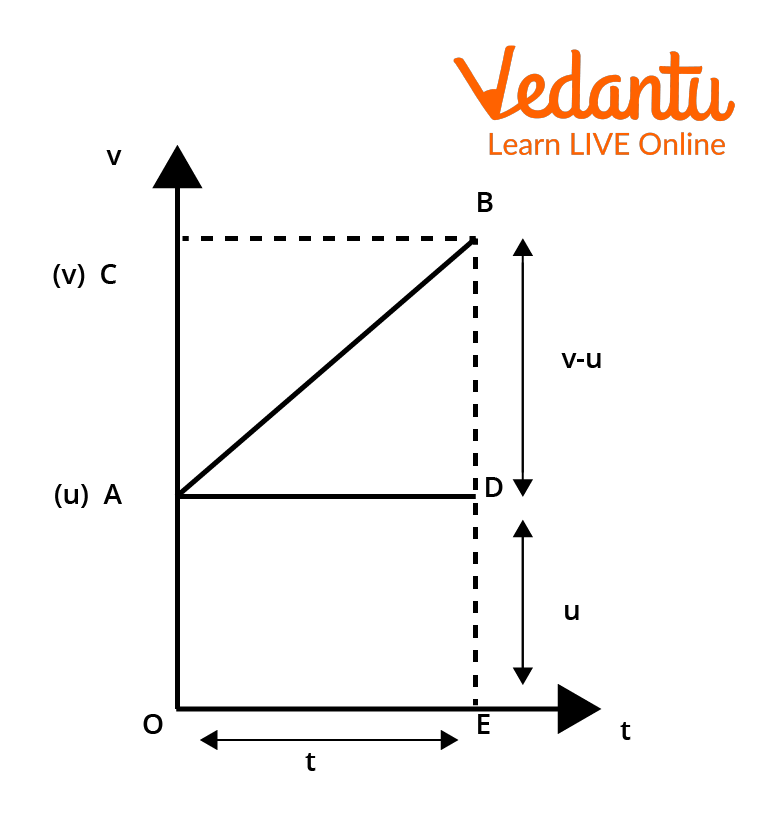Courses
Courses for Kids
Free study material
Offline Centres
More

# Graphical Methods of Vector Addition with Solved Examples for JEELast updated date: 04th Dec 2023
Total views: 23.1k
Views today: 1.23k## Introducing Graphical Methods for Vector Addition

Let’s get some insights into what is a vector. In Maths or Physics, any quantity which has only magnitude is called a scalar. If the quantity is having both the magnitude and the direction, then it is stated as a Vector. In essence, a vector is represented by an arrow having a head and a tail whose length represents the magnitude and the tail → head represents the direction of the vector. Apart from the methods discussed to make the vector addition algebraically like the law of vector addition, we also have graphical methods to adopt for those operations.

## What is a Graphical Method?

Graphical Methods are ways of finding the solution through graphs rather than solving it in algebraic ways. Vectors are drawn in a coordinate plane separately following a particular process and are added thereby measuring the length of the resultant giving the magnitude of the resultant vector. In the graphical method of vector addition, we use the number line to plot away all the vectors in a one-dimensional plane. If we are adding several vectors in a one-dimensional plane, we plot them on a number line in a stacked manner and the resultant sum of these vectors could be described as the vector that starts from the first vector and ends at the last one.

In the two-dimensional plane, we use a method called the Head to Tail Method to add vectors.

Head to Tail, as the name describes that head is the beginning of a vector arrow whereas the tail is the end part of a vector arrow. Let’s delve into the process of how we add vectors in this graphical method.

Let’s discuss all the steps that are followed in a head-to-tail process in detail.

To plot the vectors in a graph, let us assume an example of a car driving in the East direction for 4 km and taking a turn towards the North to drive for 3 km. Let us find the resultant displacement of the car through plotting in the graph.

Assuming the X-axis as the West-East Direction, and the Y-axis as the North-South Direction. Let’s follow the below-given steps to plot the vectors on the graph.

Step 1: Draw an arrow that represents the distance travelled by car towards East Direction, i.e., on X-an axis with the length of 4 units.Step 2: Draw another arrow which shows the distance travelled by car towards North Direction with the length of 3 units. Here, the tail of this arrow touches the head of the first arrow.This is called the Head to the Tail method and this process will be followed irrespective of any number of vectors being added.

Step 3: After completing the plotting of all the vectors to be added, let’s find the resultant displacement vector by joining the tail of the first vector and the head of the last vector. To find the magnitude of the final displacement, we can measure the length of the resultant vector with a ruler as shown in the figure.Head to Tail Method for Graphical Method

We can add any number of vectors in any order by following this process. This method is beneficial in the graphical addition or subtraction of vectors.

## Third Equation of Motion by Graphical Method

Let’s derive the third equation of motion using the graphical method of vector addition. Let us assume a car is moving with the initial velocity ‘u’ and final velocity ‘v’ within a period of ‘t’ secs. Also, let ‘s’ be the total distance travelled by car in t secs. If the car is travelling with a uniform acceleration ‘a’, then let’s start demonstrating this situation on the graph as shown below.Third Equation of Motion

This is a graph drawn between velocity and time wherein a car is travelling with a uniform acceleration along the path AB. Here, OA is the initial velocity and OC is the final velocity. The car has travelled from point A to point B. The distance covered by the car in t secs is given by the area of the figure OABDE. To find this area, we can divide the figure into a triangle and a rectangle.

The area of the figure OABDE = Area of the triangle ABD + Area of the rectangle OADE

The area of the rectangle OADE  = length x breadth = u.t

Area of the triangle ABD = $\dfrac{1}{2} \times \text { base } \times \text { height }=\dfrac{1}{2} \times t \times(v-u)=\dfrac{(v t - u t)}{2}$

Therefore, total area of the shape OABDE,

i.e., ${s}=u t + \dfrac{v t - u t}{2} = \dfrac{2 u t + v t - u t}{2} = \dfrac{v t + u t}{2} = \dfrac{1}{2} \cdot(v + u) t$

This is the value of distance s from the graph.

As we know that acceleration is the rate of change in velocity over time. So, this can be written as

$a=\dfrac{v-u}{t} \\ t=\dfrac{v-u}{a}$

Substituting the value of t in the distance equation, we get

$s=\dfrac{1}{2} \cdot(v+u) \cdot \dfrac{v-u}{a} \\ \\ 2 a s=(v+u)(v-u) \\ \\ 2 a s=v^{2}-u^{2}$

Therefore, the third equation of motion is obtained as follows:

$v^2=u^2+2as$

Let us solve an example using the head to tail method.

Example:  A person is walking towards the west for 7 metres and turns to his left and walks 5 metres in the south direction. Find the magnitude of the resultant displacement he has travelled using the head to tail method.

Ans:

Step 1:  Draw an arrow that represents the distance travelled by the person towards the west direction, i.e., on the negative X-axis with the length of 7 units.Step 2: Draw another arrow which shows the distance travelled by a person towards the south direction with the length of 5 units. Here, the tail of this arrow touches the head of the first arrow.Step 3: After completing the plotting of all the vectors to be added, let’s find the resultant displacement vector by joining the tail of the first vector and the head of the last vector.To find the magnitude of the resultant vector, just measure the line that is drawn in the final step using a scalar. That gives the result using the graphical method to add any number of vectors.

## Conclusion

Therefore, the graphical method of vector addition can be used in numerous applications in Mathematics like the law of vector addition. Hence, the head to tail process is explicitly explained and also the third equation of motion by the graphical method is derived. These graphical methods are also used in the subtraction of vectors. The results must be accurate and precise in this method as we measure them by ourselves. Only vectors of the same type and nature can be added together.

## FAQs on Graphical Methods of Vector Addition with Solved Examples for JEE

1. Can a vector in one dimension be added arithmetically?

Yes, the two vectors which are in one dimension could be added arithmetically because they don’t have components in any other direction except in a particular direction, an X-axis or a Y-axis, whereas the vectors that are having two dimensions can’t be added in this way. If a vector in a 2-D plane is divided into components, it will get two, one through X-axis and one through Y-axis. The respective components are added to get a respective component of the resultant. This is why they are added geometrically.

2. What is the use of the analytical method in solving vectors?

Analytical methods in vector addition use simple trigonometric formulas and the Pythagorean theorem to find the resultant of two vectors. The vector is resolved into its two vector components and thereby formulae are used on them to attain resultant components.

Through the analytical method, we can determine the components of the vectors, their magnitude, and direction. This method doesn’t have more extensive estimations and calculations which makes this method more accurate and concise than the graphical methods that we use to make graphs.